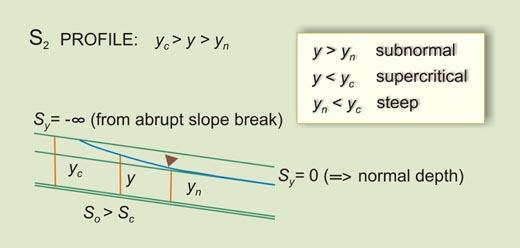online_wsprofiles_25:   S2 water-surface profileDESCRIPTION
General

• The php script [program]   online_wsprofiles_25   calculates an S2 water-surface profile in a prismatic open channel using the direct-step method (Chow, V. T.: Open-channel hydraulics, McGraw-Hill, 1959).

• The program calculates critical and normal depths based on the geometric and hydraulic input data. Newton's iteration is used to solve the nonlinear equations.

• An S2 profile is subnormal, supercritical, and steep (see figure). The calculated gradually varied flow depth varies between the specified flow depth at the upstream boundary [or, if requested, the calculated critical depth for the upstream channel] and [it is asymptotic to] normal depth downstream.

Input

• There are five required input variables:   discharge Q, bottom width B, side slope z, Manning's n, and bottom slope So.

• For the upstream boundary condition, you can specify a suitable input flow depth. Alternatively, if the input flow depth box is left blank, the program will use critical depth [in the upstream channel] instead.

• The program echoes the input data. Internally calculated critical and normal depth are echoed with five-decimal (5) accuracy.

Defaults

• For the input flow depth, you can enter any suitable supercritical subnormal flow depth. The allowable range of input flow depth is the following: Less than [or equal to] critical depth [upstream], and greater than or equal to normal depth (plus 0.002 m) downstream. Leave this box blank if you want the program to use critical depth.

• You can enter the desired number of computational intervals n. The suggested range is 50-200. A higher number means higher computational accuracy. Leave this box blank if you want the program to set the default value at 100.

• You can enter the desired number of tabular output intervals m. The suggested range is 10-100. A higher number means more detailed [longer] output. Leave this box blank if you want the program to set the default value at 10.

Output

• A table with thirteen (13) columns: output interval index k, [flow] depth (m), [flow] area (m2), velocity (m/s), velocity head (m), specific head (m), wetted perimeter (m), hydraulic radius (m), friction slope (m/m), average [friction] slope (m/m), specific head difference (m), length increment (m), and total [channel] length (m).

• The number of computational intervals n and the number of tabular output intervals m are printed for reference.

• The calculated normal depth and normal-depth Froude number are printed for reference. If the normal-depth Froude number is less than 1.02, execution is halted (See Error message No. 8 below).

Units

• The current version is written in SI [metric] units.

Errors

• Enter only positive [nonzero] numbers for discharge, Manning's n, and bottom slope (for suitable practical ranges, see error messages Nos. 4 and 5). Failure to do this will trigger an error message and halt execution (Error messages No. 1: A, B, and C, respectively).

• Enter only nonnegative [zero or greater] numbers for bottom width and side slope. Failure to do this will trigger an error message and halt execution (Error messages No. 2: A and B, respectively).

• Bottom width and side slope cannot be zero at the same time. Failure to do this will trigger an error message and halt execution (Error message No. 3).

• A Manning's n value outside of the range 0.008-0.250 will trigger an error message and halt execution (Error message No. 4).

• A bottom slope outside of the range 0.001-0.3 will trigger an error message and halt execution (Error message No. 5).

• Enter only nonnegative numbers [zero or greater] for discharge, bottom width and side slope in the upstream channel. Failure to do this will trigger an error message and halt execution (Error messages No. 6: A, B, and C, respectively). Caution: Do not specify an [actual] zero upstream bottom width Bu/s (a triangular cross section) when the downstream channel bottom width B is other than zero. Likewise, do not specify an [actual] zero upstream size slope zu/s (a rectangular cross section) when the downstream channel side slope z is other than zero. In either case, the upstream values will be redefined as equal to the specified nonzero downstream values.

• An input flow depth greater than the calculated critical depth or less than or equal to the calculated normal depth will trigger an error message and halt execution (Error messages No. 7: A and B, respectively).

• A normal-depth Froude number less than 1.02 will trigger an error message and halt execution (Error message No. 8).

Thank you for running online_wsprofiles_25.   Please call again.   [Version 1.0.0,   070502]